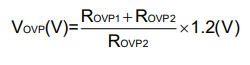# MP3362 OVP calculation

In calculating your “typical application circuit” (MP3362) for the OVP resistor values it produces very different results: 36 = (x+10000 / 10000)1.2x36=1.66K for R1(X)?

Is 1.66 the margin? Also, if I calculate a simple voltage divider circuit the OVP resistors equal 1.8V output assuming a 36V source and OVP1=294K and OVP2= 10K. What am I missing?

Thank you.

Hello Sparker,

Could you explain how you got the 1.66 margin?

Your calculation following the equation looks correct.Regards,
Vinh Tran